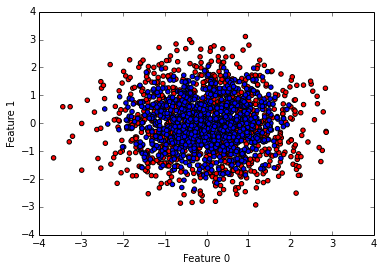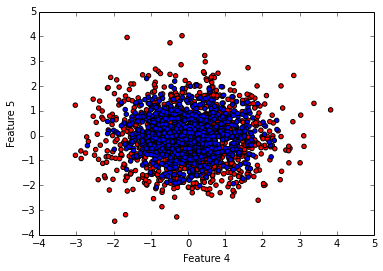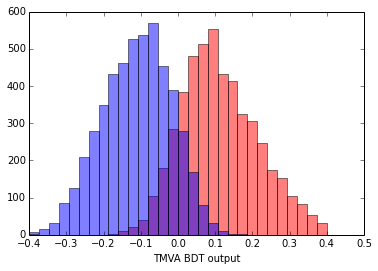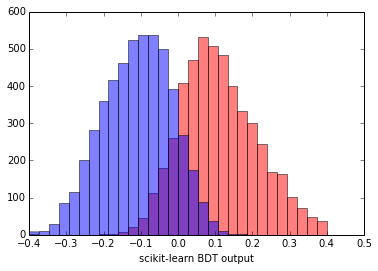# Matching Machine Learning

In High Energy Physics a lot of Machine Learning is done. Mostly with the TMVA package which is part of the ROOT framework which is used by almost every experiment. In this post I will compare how to use TMVA and scikit-learn to solve the same problem.

TMVA implements many of the popular Machine Learning algorithms, is basically the only option considered by High Energy Physicists, and is used almost exclusively by them. This means it is easy to discuss and share code with others. The fact that it is used almost exclusively by and in High Energy Physics also means it is a bit clunky to use and is missing a lot of the useful features present in other packages like scikit-learn.

Changing habits is always difficult and one of the first questions asked when you propose a change of something that works is: how do we reproduce our current results? This is how scientists adopt new strategies and techniques. You take a problem you already solved, apply a new method and check if you get the same answer. Then you apply the new method to a new problem.

Let's see if we can obtain the same result from TMVA and scikit-learn when training a set of Decision Trees combined via AdaBoost.

The first thing we need is some data to train on. As this is about AdaBoost we will use the dataset used in Hastie et al 2009, Example 10.2 where they compare the performance of a decision tree, a decision stump and a boosted decision stump.

## Creating a problem for ourselves¶

With that, some setup and data generation:

In :
%matplotlib inline

In :
from array import array

import numpy as np
import matplotlib.pyplot as plt

from sklearn import datasets
from sklearn.tree import DecisionTreeClassifier
from sklearn.metrics import classification_report, roc_auc_score

In :
# Generate data like in Hastie et al. 2009, Example 10.2.
# where DecisionTrees, DecisionStumps and AdaBoost are
# compared to each other
X, y = datasets.make_hastie_10_2(n_samples=12000, random_state=1)

# Train on the first 2000, test on the rest
X_train, y_train = X[:2000], y[:2000]
X_test, y_test = X[2000:], y[2000:]

# some shortcuts to select "signal" or "background"
# entries in the feautre vector
signal = y_train > +0.1
background = y_train < -0.1


Time to examine some of the features we have to play with. The Hastie dataset contains ten features, each of which is a unit gaussian centered on zero. A sample is considered a "signal" if $\sum_j^{10}X_j^2>9.34$. In words: if the sum of the square of each feature is larger than 9.34 it is a sample we want to keep, otherwise get rid of it. In general ML terms, samples with $\sum_j^{10}X_j^2>9.34$ form one class and all others form the second class.

Can we see anything by plotting one of the features against another?

In :
plt.scatter(X_train[signal,0], X_train[signal,1], c='red')
plt.scatter(X_train[background,0], X_train[background,1], c='blue')
plt.xlabel("Feature 0")
plt.ylabel("Feature 1")

Out:
<matplotlib.text.Text at 0x10c90cc10>In :
plt.scatter(X_train[signal,4], X_train[signal,5], c='red')
plt.scatter(X_train[background,4], X_train[background,5], c='blue')
plt.xlabel("Feature 4")
plt.ylabel("Feature 5")

Out:
<matplotlib.text.Text at 0x10ca56dd0>Not really. There does not seem to be a clear pattern on how to separate blue from red points by looking at any pair of variables. Good luck Mr DecisionTree!

## Training a BDT with TMVA¶

First we will train the default Boosted Decision Tree classifier in TMVA. After this we will try and replicate this with scikit-learn.

In order to use TMVA we have to create a Factory object, to which we add the individual samples, labelling them as either "signal" or "background" and as "testing" or "training".

In :
import ROOT as R
from ROOT import TMVA

# ROOT and TMVA require an open file to store things
# as they go along
outfile = R.TFile('/tmp/tmva_output.root', 'recreate')

factory = TMVA.Factory("TMVAClassification", outfile, "AnalysisType=Classification")
for n in range(10):

for y,row in zip(y_train, X_train):
a = R.std.vector(R.Double)()
for r in row:
a.push_back(r)

if y > 0:
else:

for y,row in zip(y_test, X_test):
a = R.std.vector(R.Double)() # instantiate a std::vector<double>
for r in row:
a.push_back(r)

if y > 0:
else:

factory.PrepareTrainingAndTestTree(R.TCut("1"), "SplitMode=Random:NormMode=NumEvents")


After adding each sample we can now set what kind of classifier we would like to train as well as the hyperparameters for that classifier. After that we tell TMVA to go and train the classifier.

In :
factory.BookMethod(TMVA.Types.kBDT, "BDT",
#"NTrees=800:nEventsMin=100:MaxDepth=3:"
"nCuts=-1"
)
factory.TrainAllMethods()


The options (or hyperparameters) of the BDT are all left at their default values. If you were to uncomment the long option string, nothing would change. The only thing we need to adjust is nCuts which determines how many "steps" TMVA will take when evaluating where to split a feature when building a new leaf. By setting it to -1 it will evaluate "every possible" cut value instead of splitting the range of a feature into N chunks.

As part of the training TMVA creates a "weights" file called weights/TMVAClassification_BDT.weights.xml. This is where all the information about the trained classifier is stored. In order to access the classifier we just trained we need a so called Reader object. The idea behind this split of training and using the classifier is that you typically train the classifier once and then use it many times in different applications. As part of that we have to redeclare all the features we wish to use:

In :
reader = TMVA.Reader()
for n in range(10):
a = array("f", [0.])

y_predicted = []
decision_val = []
for row in X_test:
a = R.std.vector(R.Double)()
for r in row:
a.push_back(r)

decision_val.append(value)
if value > 0:
y_predicted.append(+1)
else:
y_predicted.append(-1)

print classification_report(y_test, y_predicted, target_names=["background", "signal"])
print "Area under ROC curve: %.4f"%(roc_auc_score(y_test, y_predicted))

             precision    recall  f1-score   support

background       0.87      0.89      0.88      5046
signal       0.88      0.87      0.88      4954

avg / total       0.88      0.88      0.88     10000

Area under ROC curve: 0.8783


Above you can see various performance metrics evaluated on the test set for the BDT trained by TMVA. We also print the area under the ROC curve.

In High Energy Physics people like seeing the shape of the BDT output distribution. Afterall seeing is believing! You can see there is very little overlap between the red and blue. This is good, it means we can use the BDT output to separate our two samples.

In :
D = np.array(decision_val)
plt.hist(D[y_test>0.],
color='r', alpha=0.5, range=(-0.4,0.4), bins=30)
plt.hist(D[y_test<0.],
color='b', alpha=0.5, range=(-0.4,0.4), bins=30)
plt.xlabel("TMVA BDT output")

Out:
<matplotlib.text.Text at 0x1128bfc10>## Next up scikit-learn!¶

Now for doing the same in scikit-learn! We setup the weak learner used by AdaBoost with the same options as used in TMVA, then train it and evaluate the same performance metrics, as well as the area under the ROC. The performance metrics are almsot exactly the same, and the area under the ROC is exactly the same (within the precision of the print out).

In :
dt = DecisionTreeClassifier(max_depth=3,
min_samples_leaf=0.05*len(X_train))
algorithm='SAMME',
n_estimators=800,
learning_rate=0.5)

bdt.fit(X_train, y_train)
sk_y_predicted = bdt.predict(X_test)
print classification_report(y_test, sk_y_predicted, target_names=["background", "signal"])
print "Area under ROC curve: %.4f"%(roc_auc_score(y_test, y_predicted))

             precision    recall  f1-score   support

background       0.88      0.88      0.88      5046
signal       0.88      0.87      0.88      4954

avg / total       0.88      0.88      0.88     10000

Area under ROC curve: 0.8783

In :
plt.hist(bdt.decision_function(X_test[y_test>0.]).ravel(),
color='r', alpha=0.5, range=(-0.4,0.4), bins=30)
plt.hist(bdt.decision_function(X_test[y_test<0.]).ravel(),
color='b', alpha=0.5, range=(-0.4,0.4), bins=30)
plt.xlabel("scikit-learn BDT output")

Out:
<matplotlib.text.Text at 0x111906890>Compare the above figure of the BDT output from scikit-learn with the one we got from TMVA. They are remarkably similar! With such similiar BDT responses we can be pretty confident we managed to replicate what TMVA does with scikit-learn.

So now that we understand how to configure scikit-learn to reproduce previous results, we can go forth and fiddle with the tools that it provides. The scientific method is as much about taking small steps where you can predict what will happen as huge leaps which will turn out to be great discoveries!

This post started life as a ipython notebook, download it or view it online.